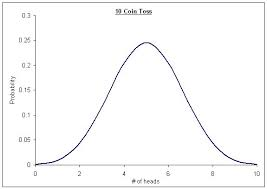## How to Calculate and Solve for Certainty | ProbabilityThe image above represents Certainty.

To compute for certainty, one essential parameter is needed and this parameter is Total Number of All Possible Outcomes (N).

The formula for calculating Certainty:

P(A) = x N = N N

Where;

P(A) = Certainty
N = Total Number of All Possible Outcomes = 2

Let’s solve an example;
Given that the total number of all possible outcomes is 2. Find the Certainty?

This implies that;

N = Total Number of All Possible Outcomes = 2

P(A) = x N = N N
P(A) = 2 2
P(A) = 1

Therefore, P(certainty) is 1.